# Introduction

1. Showing that the pure data driven approach, often blindly used in empirical data science, is not offering satisfying results because models are treated as ready-to-use black-boxes whose parameters are just mathematical proxies to optimize a loss function without any concrete reality¹.
2. Providing an example of methodology that has few realistic and testable hypotheses, and allows to introduce essential expert knowledge to improve the pure mathematical model as well as properly testing the model behavior.

We are interested by modeling the number of detected cases. The reason why we are interesting specifically in the number of detected cases is that it seems to be the easiest quantity to model. Once we have a solid model for the number of detected cases, we can derive others quantities like number of deaths, estimation of real infected, etc.
The very first hypothesis we will make is that (H₀) for a given relatively homogeneous geographical location, the evolution of the epidemic follows an unknown stochastic process. By relatively homogeneous, we mean that it holds for a country, or a state for large countries such as US or China, but might not hold for instance for an area covering Belgium and Netherlands as the national level policies are not the same, nor the behavior of the population in general and w.r.t. social distancing measures.

# Setting up the problem and hypothesis

So if we take the current time series available for one particular country, e.g. Italy, it corresponds to the beginning of ONE single trajectory. It is thus difficult not to say impossible to draw any conclusion on the stochastic process itself. To do so, we would need to have many copies of Italy and restart the exact same epidemic several times, which is obviously impossible. This is one of the most common and major pitfall of data science: it is sometimes difficult to know if the time series we have come from the same stochastic process or are just trajectories for different processes. It is very tempting to consider the underlying process is the same for all trajectories but fundamentally (epistemologically I would even say) wrong. In this paper, we will try to avoid this pitfall.

Let’s make as few hypothesis that we can, and let us ensure they are plausible and testable.

## Hypothesis H₁

In this article, we use the term sigmoid to define any function that has a point of inflection such that the function is convex before and concave after. This is more restrictive than the correct mathematical definition but it is enough to serve our purpose. Also, we assume the sigmoid is symmetric around its inflection point. The method would world with non symmetric but would had an additional parameter to estimate. We leave this for future work.
Therefore, the typical sigmoid prototype is described by the following:

where a is a scaling factor, λ the growth rate and t_λ the time at midpoint (i.e. at the point of inflection). In a normalized state space, i.e. for a=1, t_λ=0, the only free parameter is the growth rate as illustrated by Figure 1.

The rationale behind this hypothesis is that, the number of cases will necessarily reach an asymptotic number: either the whole population, or a fraction of it. The fraction could a function of the virus characteristics, people developing immunity, effectiveness of social distancing, etc.

The noise is a random variable to model the day by day variation due to several possible factors that are hard to estimate or require expert knowledge (e.g. number of tests performed, number of people that decided to go to the hospital to take the test, or a new way of counting infected people as it happened in China, etc.). In this paper, we consider that the noise is iid but, as pointed out by Redha Moulla, it is more likely to behave as a Brownian motion with drift. For instance, for France, the trend of the number of tests performed is definitely not constant as illustrated by Figure 2 (top). However, we leave this improvement for future work. Actually, it is enough to talk few minutes with the experts to know that, indeed, the testing strategy highly impact the result as illustrated by Figure 2 (bottom).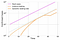Figure 2. Top: Cumulative number of tests performed by France depending on the week. Bottom: Influence of testing strategy on the number of detected case.

Finally, this hypothesis can be tested on all the previous epidemics recorded by humanity, and models made by epidemiologists (e.g. advanced SIR-based models).

Hypothesis (H₁) implies that, all we have to do is to look for the parameters of a sigmoid:

1. λ: the growth rate,
2. t_λ: time at the midpoint,
3. a: asymptotic number of detected cases (capacity),

A common approach in data science would be to estimate these parameters by fitting a sigmoid with a RMSE loss. Why it does not work? For two reasons:

1. there is no empirical or “physical” justification for the estimation of these parameters. What is the rationale to support that the estimation of t_λ through a simple RMSE minimization is actually the real peak in terms of new detected case per day? Or more concretely: I can perfectly fix t_λ for any reason, and fit the curve. Most like, the result for a and λ will be much different but no one will be able to see a reasonable difference between the two estimated curve when it comes to compare on the available data (the time series up to today). What I just do when fitting, is using a mathematical proxy in case I do not have a close form on my model.
2. more fundamentally, the available data represent only one trajectory. Even if the fit was perfect, it does tell nothing about the underlying stochastic process for the given country, and obviously even less when it comes to transfer this knowledge to other countries.

So let us explicit (H₁) a bit further:

1. for a given country, λ, t_λ and a are unknown. However, we need to know only λ and t_λ, because a can be deduced as 2x_{t_λ} by the properties of a sigmoid. These parameters are proper to a given location and each of them are noisy.
2. Let’s consider N countries, and the vector of stochastic processes {Xₜ}ₜ = (X¹ₜ, …, Xᴺₜ), where Xⁱₜ ∼ Sₜ(λⁱ, t_λⁱ) + εⁱₜ.

Due to many factors depending mostly on the country, the value of λⁱ and t_λⁱ are different for each i ∈ [1, N].
However, we can easily assume that there exists a matrix Φ = [ Φᵢⱼ]ᵢⱼ where Φᵢⱼ = Id, Φᵢⱼ is unknown for i < j, and Φᵢⱼ = Φᵢⱼ⁻¹ for i > j such that the processes are calibrated (i.e. Xᵢ ∼ Xⱼ). In other words, there exists an invertible mapping that basically allows to calibrate one process on another. And in particular, aligning the trajectories.

Aligning sigmoids is fairly easy in the case of symmetric sigmoids and we provide simply an illustration to explain the effect of Φ. Of course, aligning partial trajectories leads to potentially more errors. There is most likely a closed form to express the transformation and quantifying the error, but due to the time constraint, we chose to minimize the RMSE. Notice that this is not at all the same as fitting a curve to obtain “prediction”: the optimizer is used as a tool to solve a purely technical and mathematical problem, not to answer our initial problem. For more info about sigmoid calibration, we refer to the introduction provided by scikit-learn documentation. It concerns the calibration of classifier but the mathematical idea is the same.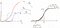Figure 3. Illustration of trajectory calibration. Left: three trajectories aligned on an arbitrary t₀. The dashed points on the left represents the date up to which we know the trajectory. Right: result of applying Φ on each trajectory. The normalized trajectory (in black) has a growth factor deduced from the reference trajectories.

A last thing is, for pandemic, the stochastic process for the whole world is the sum of stochastic processes for each countries with a potential time offset between the variables.

The sum of sigmoids is not a sigmoid. However, as the derivative of a sigmoid is approximately a Gaussian, and as the sum of Gaussian is a Gaussian, we can assume the sum of sigmoid approximately behaves like a Gaussian. This can be roughly validated empirically by looking at the time series so far. Therefore, the parameters of the global process is given by a = ∑ᵢ aⁱ and λ = √(∑ᵢ (λⁱ)²). More details on the approximation can be found at here. A more general note on the sum of sigmoids can be found here.

The approximation works well when the points of inflection are aligned. It is more or less true for COVID-19 pandemic at the exception of China as illustrated on Figure 4.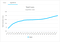Figure 4. Taken globally, the total number of cases does not look like following a sigmoid nor having an obvious exponential shape. This is due to China’s epidemic trajectory that is over. Removing it leads to a perfect exponential growth.

This approximation is a possible way to check our model: if we made correct estimations for all the parameters of each processes, we should observe that the global number of infected (minus China) follows a sigmoid with the parameters given above.

## Hypothesis (H₂)

Social distancing takes different forms and has obviously different impact depending on how it is followed by people and many other factors. Let’s denote t_Lⁱ the date social distance or lockdown has been acted (in practice it often came with more and more restrictive measures but this is something that can be adjusted later with expert knowledge). This implies that the random variable t_λⁱ depends on the known information. Let us assume then that t_λⁱ = f(t_Lⁱ) where f is an unknown measurable map. In other words, one of the two values we would like to estimate now depends (partly) on data available as of today for most countries. More concretely, it means that the point of inflection is a function of (at least) the moment social distancing measures are in place.

# Estimating the parameters

First of all, let us consider a reference trajectory, in our case Hubei. We can normalized it on [-∞, +∞] × [0,1] such that the inflection point is (0, ½). Let’s denote this trajectory x⁰ and align all trajectories on this one. For the sake of readability, we work from now on only in this normalized space, but it is important to keep in mind we have a collection Φ₀ᵢ⁻¹ of transformations to switch back to the original space. Therefore, we can consider a single process X with N (potentially partial) trajectories xₜ = {xₜⁱ}ᵢ.

As we do not have any knowledge about the map f (experts would certainly be able to define a proper model), we assume that t_λ is a RA with a Gaussian law of unknown parameters m and σ. Without loss of generality, let’s assume that the trajectories xₜ are sorted by the time they reach their peak of maximal number of new cases per day, and that J ∈ [0, N] represents the number of trajectories that reached the peak as of today.

Therefore, we can build standard indicators for m and σ based on the first J trajectories, under the assumption J > 0.

For the mean, we use the classic unbiased estimator:

For the variance, we need at least J > 1, and we use a non-biased estimator that depends on J.
Using Cochran’s theorem, we can calculate the correction to eliminate the bias from the naive standard deviation estimator:

For instance, assuming that two trajectories reached their peak, the unbiased estimator σ̅² is given by

This correction is important because the bias of the estimator depends on the number of trajectories and is obviously higher with few of them as illustrated by Figure 5.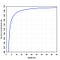Figure 5. Bias on naive variance estimator depending on the sample size.

If we have only one full trajectory, we can fix as a prior σ to 1 or 2 or 3 and observe different scenarios with a given α risk (e.g. 0.05).

Three remarks:

1. Contrarily to most basic models we have seen, we use ALL information available as of today.
2. If t_λ really follows a Gaussian, then with time, we will be able to have a better estimation and adjust all trajectories predictions.
3. If t_λ does not follow a Gaussian, then we have two choices:
1. without expert knowledge: by looking at the distribution, we might have a better understanding of the underlying law (e.g. Cauchy or Tucky-lambda) and thus, adjusting our assumption and estimators.
2. with expert knowledge: the experts might create a model f to model the influence of social distancing on the date of the inflection point. Of course, it is not an exact science, such that we can still assume the Gaussian distribution as sum of iid RV. The difference lies in the interpretation of the Gaussian: in the first case it is a naive model for f used as a prior, in the second case it models the error on f.

The useful trick to switch from the naive approach to the expert knowledge approach is the transfer theorem:

This allows to add expert knowledge at any moment to improve further the model. Some ideas to model social distancing effects can be found here. On top that, as suggested by Redha Moulla, people would stick to drastic measures as long as the figures are perceived as bad, and be more relaxed at the beginning and the end. We could test this hypothesis using a simple toy-model for f following U shape in time. We leave this for future work.

So far we have an estimator for t_λ as long as we have at least one country with a full trajectory. The only thing left is an estimator of λ that is to say, the growth rate.

Let’s try to illustrate the method on a simple example.
First, as we assumed that we have at least one full trajectory available (it is the case thanks to Hubei), the real value for t_λ¹, and x_{t_λ}¹ (the value for the first trajectory at the point of inflection) are known.
We can use a sigmoid regression with a RMSE loss to find λ¹ while the other two parameters are known with precision. We can do the same for each trajectories already completed. Note again that this is different that using a sigmoid model to make predictions since here, we have the full trajectory and we know that it behaves like a sigmoid.

We can thus estimate the expected λ of the aligned stochastic process. Two choices:

1. Conservative choice: we use only the full trajectories to estimate the λ and the estimator is the average of the λⁱₜ for each i from 1 to J.
2. YOLO choice²: we use a pondered sum for the estimator:

It requires to fit for every trajectories to this point. As the trajectories are aligned and normalized, we can take the empirical value to the latest known point for each trajectory. For full trajectories, ωᵢ = 1. The rational behind this is illustrated Figure 6.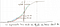Figure 6. If the trajectories calibration was done properly, ωᵢ represents how much we know about xᵢ under the assumption that the sigmoid is symmetric.

In the examples of the final model, we used only the first choice.

Finally, as we know the transformations Φ₀ᵢ, we can deduce the real parameters for each trajectory. Each of them are estimated using the whole available information.

Let us summarize the model in few steps:

1. Take the full trajectories available as today.
2. Normalized arbitrarily one of them in [-∞,+∞] × [0,1] such that the point of inflection is (0, ½).
3. Find the {Φ₀ᵢ}ᵢ that align the partial trajectories on the reference (normalized) one, either by closed form or using some optimization program as we did (it is not just scaling!).
4. Estimate the expected point of inflection based on the full trajectories and the date and effect of their social distancing measures.
5. Find the expected λ by the best fit that must go through the estimated point of inflection, either on the full trajectories, or over all trajectories by pondering by the empirical value of the trajectory as of today.
6. Use {Φ₀ᵢ⁻¹}ᵢ to go back to the initial space.
7. Project using the estimated values.

# Results and Illustration

1. we provide three scenarios: central, worst case at 5% and best case at 5\% (under a Gaussian assumption on the noise and on the uncertainty on estimation of t_λ). When the real curve starts to deviate from the central scenario, we readjuste the individual trajectory parameters to realign the central scenario on it. This affects positively all other trajectories.
2. we provide and monitor the absolute and relative uncertainty between the 5% scenarios and the average scenario to ensure it converges toward zero with time.

We found an average relative error for the central scenario for a J+1 prediction from 28/03/2020 to 01/04/2020 as follows:

1. Italy: 1.14%
2. Poland: 1.35%
3. Portugal: 1.73%
4. France: 2.08%

The detailed day by day predictions for a J+1 prediction are provided below.

`Francei Date Pred Real Error66 28/03/2020 36721 37575 2.2767 29/03/2020 41351 40174 2.9368 30/03/2020 46294 44550 3.9169 31/03/2020 51508 52128 1.1970 01/04/2020 56938 56989 0.09Italyi Date Pred Real Error66 28/03/2020 92079 92472 0.4267 29/03/2020 97699 97689 0.0168 30/03/2020 103033 101739 1.2769 31/03/2020 108036 105792 2.1270 01/04/2020 112676 110574 1.90Portugali Date Pred Real Error66 28/03/2020 5090 5170 1.5467 29/03/2020 5876 5962 1.4468 30/03/2020 6663 6408 3.9869 31/03/2020 7422 7443 0.2770 01/04/2020 8130 8251 1.46Polandi Date Pred Real Error66 28/03/2020 1603 1638 2.0867 29/03/2020 1832 1862 1.5668 30/03/2020 2076 2055 1.0269 31/03/2020 2329 2311 0.7870 01/04/2020 2587 2554 1.29`

We illustrate the current model predictions on data up to 2020/04/01 in the Figures below.

# Discussion

Some drawbacks of this approach:

1. we need to have at least a full trajectory as reference. Technically we could do it with only partial trajectories but we doubt it would lead to good results.
2. it works only for countries that established social distancing measures.

1. we have only two testable and rather obvious hypotheses,
2. there are few parameters to estimate that are by construction in relation with “physical” quantities available as of today (social distance date and effect).
3. the model uses all the available information at time t, even partial trajectories, contrarily to model that simply fits naively a curve.
4. we know it converges and we can get reasonable estimation using concentration inequalities.
5. the model allows different scenarios for a different α risk thanks to the probabilistic modeling. In particular, the key point is the estimation of the point of inflection depending on the day of introduction of social distancing measures and their impact. As a naive approximation, we assumed it follows a Gaussian such that we have a closed formed for the confidence interval. For more complex distributions or model for f, we can simply sample to deduce the scenarios for any risk level.
6. we can check many things:
1. if the trajectories are outside of the confidence interval (at e.g. 5%), it is most likely the model estimations that are wrong rather than a country behaving differently than the others. Therefore we can adjust manually or automatically the bias on the impact of social measures.
2. if the global trajectory correctly follows the trajectory induced by the estimation for each individual trajectories (cf. the note on the sum of sigmoids). The observed relative errors indicates the direction of the bias in our estimator for t_λ and λ and a hint on how big this bias is.
7. we can make a lot of counterfactual analysis:
1. the deviation of individual trajectories depending on the social distancing date,
2. the deviation of the trajectory by removing T dates in the available time series for a given country,
3. the marginal influence of each country in the estimated model parameters.

From this model, we can then study further questions:

1. what is the distribution of real cases, knowing the virus characteristics (incubation, ratio of asymptomatic), test policy, etc.
2. given characteristics about the healthcare systems, what is the choke point that will translate into over-mortality (people that died because they could not receive proper treatment due to medical equipment shortage) and until when over-mortality occurs and with what effect on the asymptotical death rate.

There are several possible improvements as mentioned all along this article. We believe that it gives at least an interesting methodology with a sound founding that can be easily improved by inserting expert knowledge, notably on the social distancing measure impact as well as the report methodology that may vary in time. Also, although the hypothesis on sigmoid works well in vitro, but in vivo, with the potential of epidemic resurgence or future epidemic waves, the sigmoid might not be adequate and taking examples on e.g. seasonal flu and other epidemiological knowledge would for sure be useful.

Again, we are not epidemiologist and the point if this modest article was only to show that it is possible to create purely mathematical models that can leave a door open to expert knowledge. The pure mathematical framework is only a tool and not the perfect representation of reality: it is not because, e.g. we know that an estimator is the Best Linear Unbiaised Estimator (BLUE) that using this estimator makes sense or will give the real optimal result for our practical problem. In other words, expert knowledge is the most important, and a solid mathematical modeling skill is just a (necessary) medium. This is, we believe, the main pitfall of the current data science and AI industry.

A last fun fact: if China would have lied about the number of cases in Hubei, the model proposed here would not work. Or at least, it would introduce an enormous bias until enough countries reach their peak of new cases per day. To be more precise, it could work if they planned to communicate figures such that it follows a sigmoid. But even though, the growth rate would be so different that I doubt it would work.

# Acknowledgment

Of course, I need to warmly thank Sławomir Kumka, head of IBM Poland R&D Lab, who was the first to challenged me to come up with something about COVID19. Finally, this would not have been possible without the time granted both by the local management and the global IBM program #FridayYOU that gives Friday afternoon to all employees to develop their skills or to volunteer.

1: Sounds harsh, I know… but I really do believe this is one of the main factors why so many Data Science and AI projects fail.

2: aka I do not have the convergence proof yet.

Written by

Written by

## Alexandre Quemy

#### Engineer at IBM, PhD candidate in AI at Poznan University of Technology